# Reach for the Summit problem set - Physics

Welcome to the road to Olympics! By this problem set, you will lead your way to the summit of physics, just like climbing mountain in Celeste.

To match the Celeste scheme, each section will be seperated into A,B,C sides, each of which has lots of problems, having the similar format. The difficulty of the problems may be randomly shuffled, though.

I'll use this problem set for my games or programs later on, and I will upgrade it frequently.

Problem Format: For example, if the problem is on Stage 1, A side and 5th position, then the name of the problem will be:

Reach for the Summit - P-S1-A5

Stage 1: Kinematics

Stage 2: Equilibrium of Objects

Stage 3: Newton's Laws of Motion, Inertia

Stage 4: Centey of Mass, Momentum and Angular Momentum

Stage 5: Energy

Stage 6: Vibration and Wave

Stage 7: Thermodynamics

Stage 8: Electrostatic Field

Stage 9: Constant Currents

Stage 10: Magnetic Field and Induction

Stage 11: Alternating Current and Electromagnetic Wave

Stage 12: Optics

Stage 13: Modern PhysicsNote by Alice Smith
1 year ago

This discussion board is a place to discuss our Daily Challenges and the math and science related to those challenges. Explanations are more than just a solution — they should explain the steps and thinking strategies that you used to obtain the solution. Comments should further the discussion of math and science.

When posting on Brilliant:

• Use the emojis to react to an explanation, whether you're congratulating a job well done , or just really confused .
• Ask specific questions about the challenge or the steps in somebody's explanation. Well-posed questions can add a lot to the discussion, but posting "I don't understand!" doesn't help anyone.
• Try to contribute something new to the discussion, whether it is an extension, generalization or other idea related to the challenge.

MarkdownAppears as
*italics* or _italics_ italics
**bold** or __bold__ bold
- bulleted- list
• bulleted
• list
1. numbered2. list
1. numbered
2. list
Note: you must add a full line of space before and after lists for them to show up correctly
paragraph 1paragraph 2

paragraph 1

paragraph 2

[example link](https://brilliant.org)example link
> This is a quote
This is a quote
    # I indented these lines
# 4 spaces, and now they show
# up as a code block.

print "hello world"
# I indented these lines
# 4 spaces, and now they show
# up as a code block.

print "hello world"
MathAppears as
Remember to wrap math in $$ ... $$ or $ ... $ to ensure proper formatting.
2 \times 3 $2 \times 3$
2^{34} $2^{34}$
a_{i-1} $a_{i-1}$
\frac{2}{3} $\frac{2}{3}$
\sqrt{2} $\sqrt{2}$
\sum_{i=1}^3 $\sum_{i=1}^3$
\sin \theta $\sin \theta$
\boxed{123} $\boxed{123}$

Sort by: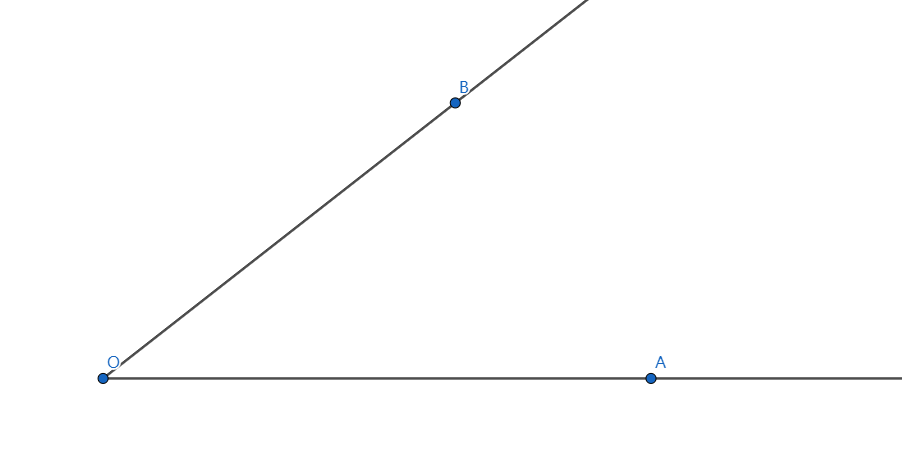A UFO is moving at a constant velocity $v$ from $O$ along ray $OB$, and $OA$ is horizontal, as shown in the picture. Alice is observing it at point $A$, and $\angle BOA = \theta$, which can be considered constant during the observation.

Given that the UFO is giving off a small pulse of sound wave and then a big pulse after $\tau\ (\tau \rightarrow 0)$ seconds.

If $OA=L$, and the speed of sound in the air is $v'$, at what condition for $v$ can Alice record the big pulse first and then the small pulse of sound wave?

Take $\theta = 30 \degree$, and the condition is $v > \lambda v'$. Submit $\lfloor 1000\lambda \rfloor$.

- 1 year ago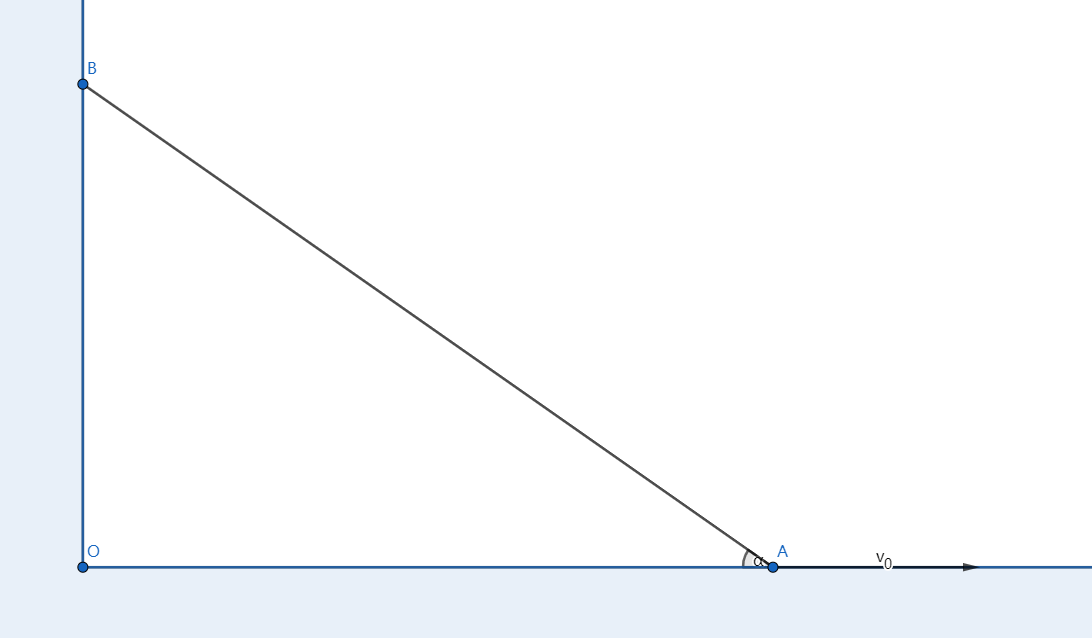As shown above, a rod with length $l$ is leaning against the vertical wall. The lowest point of the rod, $A$ is moving right at velocity $v_0$, at this time, the angle of the rod and the ground is $\alpha$.

Then there exists a point on the rod which has the minimum magnitude of velocity. Find the minimum velocity.

Take $\alpha=30 \degree$, and the minimul velocity is $\lambda v_0$. Submit $\lfloor 10000 \lambda \rfloor$.

- 1 year ago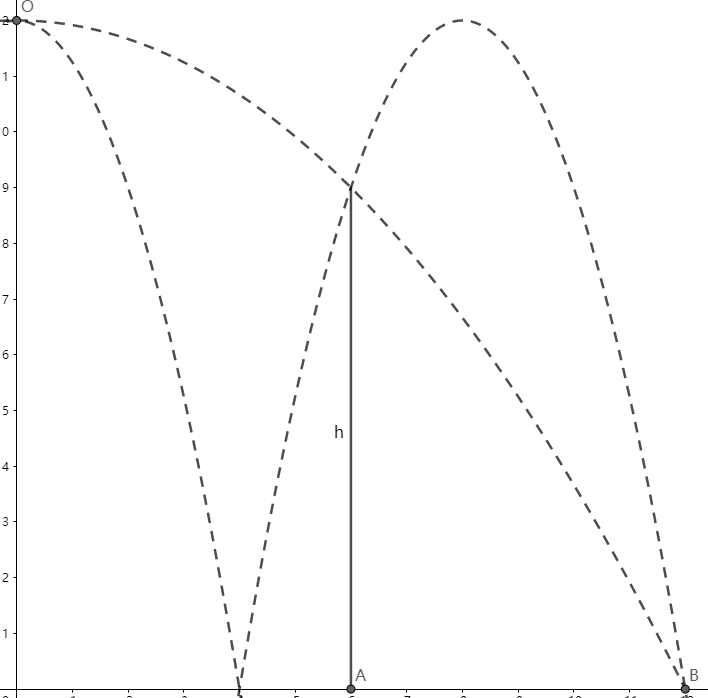As shown above, the point $O$ is $H\ m$ away from the ground. Two balls $1,2$ are thrown at point $O$ horizontally, so that ball $1$ merely passes the top of the fence and falls at point $B$, ball $2$ is bounced by the ground once and then merely passes the top of the fence and falls at point $B$.

If the collision of ball $2$ and ground is elastic, find the height of the fence $h\ (m)$.

The result is $h=\lambda H$, submit $\lfloor 1000\lambda \rfloor$.

- 1 year ago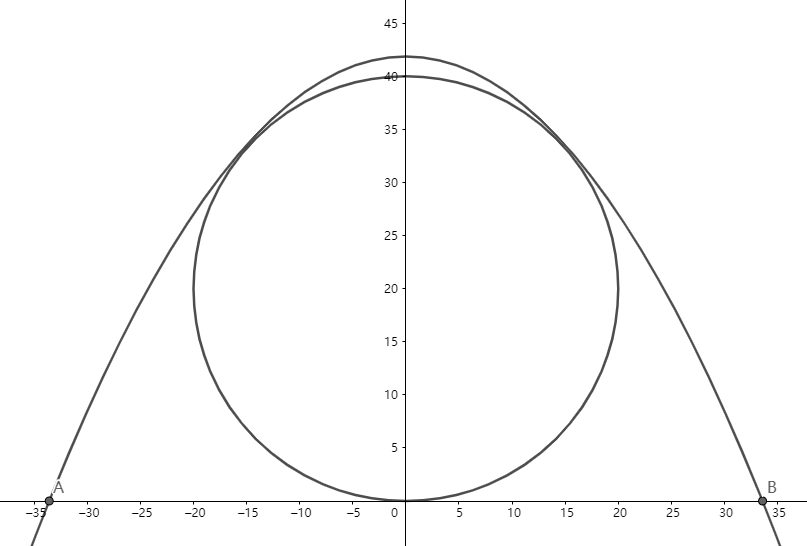A pipe with diameter $D=0.2\ m$ is put on the horizontal ground, and Ant-Man wants to jump over it to practice his strength.

If Ant-Man is initially on the ground, and he can start to jump anywhere, what's the minimum initial velocity he should have to jump over the pipe?

Let $v_m$ be the minimum velocity ($m/s)$. Submit $\lfloor 1000v_m \rfloor$.

Assumptions:

• Ignore air resistance.

• He can be treated as a mass point.

• Take gravitational acceleration $g=10 m/s^2$.

- 1 year ago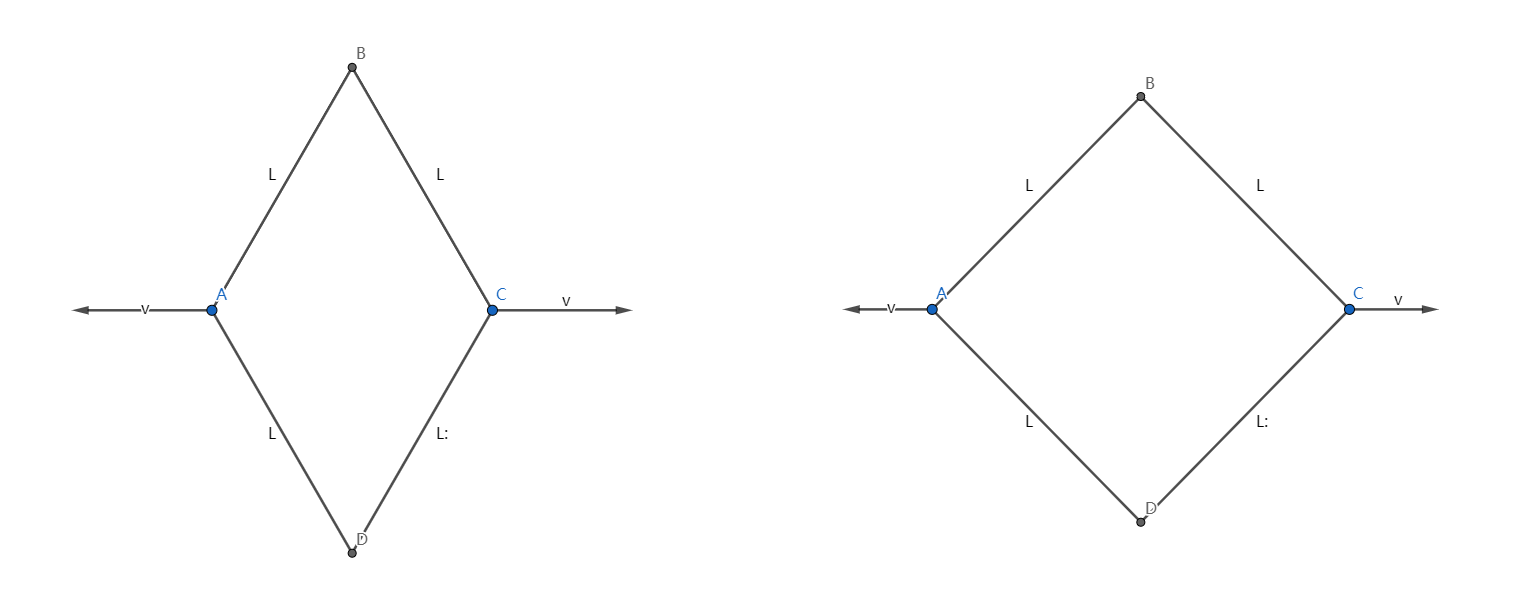As shown above, $AB,BC,CD,AD$ are four rigid rods connected by hinges whose lengths are $L$, and it's obvious that quadrilateral $ABCD$ is a rhombus.

Initially, the diagonal $BD$ is longer than $AC$, and the rhombus is put on the horizontal ground, then $A,C$ is moving to the opposite side along line $AC$ with the same magnitude of velocity $v$.

What's the acceleration $(m/s^2)$ of point $B$ relative to the ground at the moment when the rhombus becomes a square (see the picture on the right)?

Submit the value when $L=\sqrt{2}\ m,\ v=10\ m/s$.

- 1 year ago